# Spacetime (spin 1)

Speculative theories with more than one time dimension have been explored in physics. The additional dimensions may be similar to conventional time, compactified like the additional spatial dimensions in string theory, or components of a complex timeIn physics, spacetime is a mathematical model that combines the three dimensions of space and one dimension of time into a single four-dimensional manifold.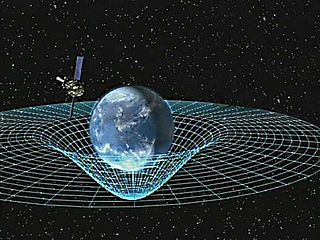Spacetime diagrams can be used to visualize relativistic effects, such as why different observers perceive differently where and when events occur.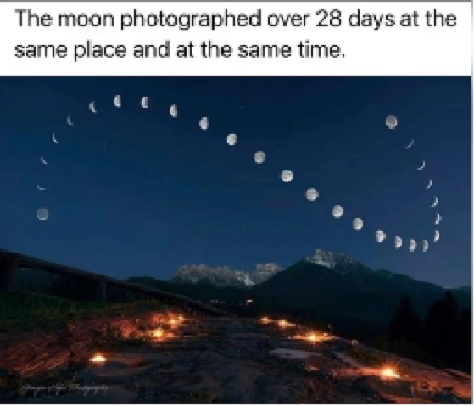Einstein's general theory of relativity, published in November 1915, describes gravity as the warping of spacetime by masses such as the Earth and moon. The latest issue of Science News celebrates general relativity's 100th anniversary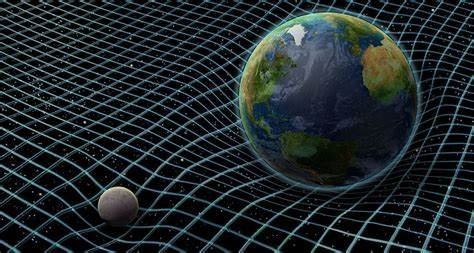The Solar System is the gravitationally bound system of the Sun and the objects that orbit the star. The largest of such objects are the eight planets. This was formed 4.6 billion years ago from the gravitational collapse of a giant interstellar molecular cloud.

Note

Zecharia Sitchin suggests that the star-shaped symbol and 11 other dots on this Sumerian cylinder seal, known as VA243, represent the sun, moon and 10 planets including a mysterious “world” known as Nibiru. How could the ancient Sumerian civilization describe our solar system so accurately if it is only possible to see five planets with the naked eye? This seems impossible if we consider the science and technology needed to observe our galaxy today. If Stichin assumptions are correct, we’ll see NIBIRU soon.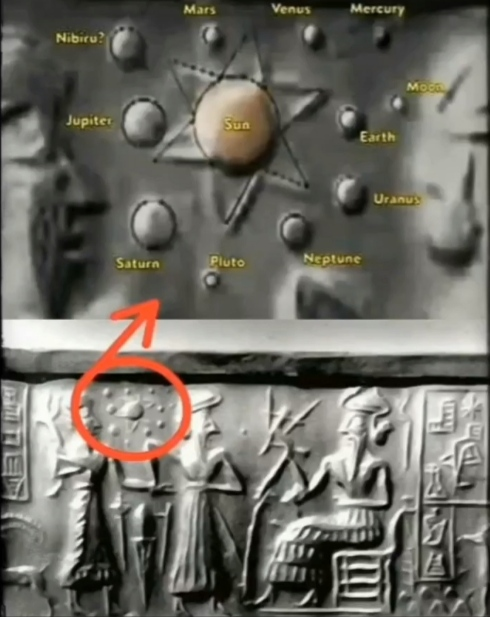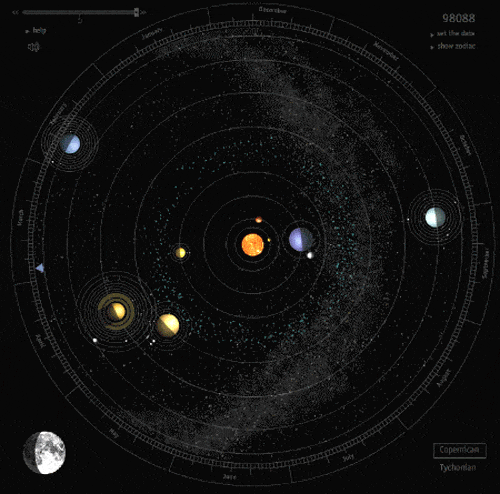Space and Time: Minkowski's Papers on Relativity, published by the Minkowski Institute. Hand-tinted transparency presented by Hermann Minkowski in his famous Raum und Zeit talk to the German Society of Scientists and Physicians in 1908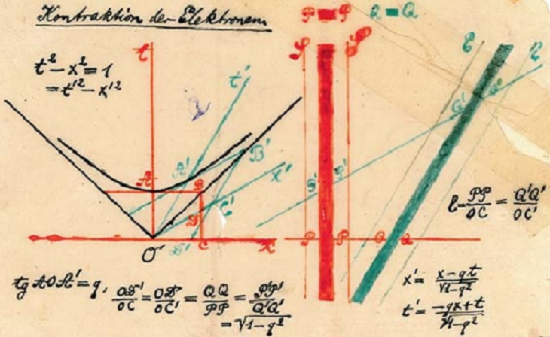Besides many theories there is COMPOSITE and PRIMES as a self organized system (12/12/12). Even though it is proven that it is not from Tesla, whoever made it if you are reading this article, I sincerely want to thank you because I use a lot of the analysis.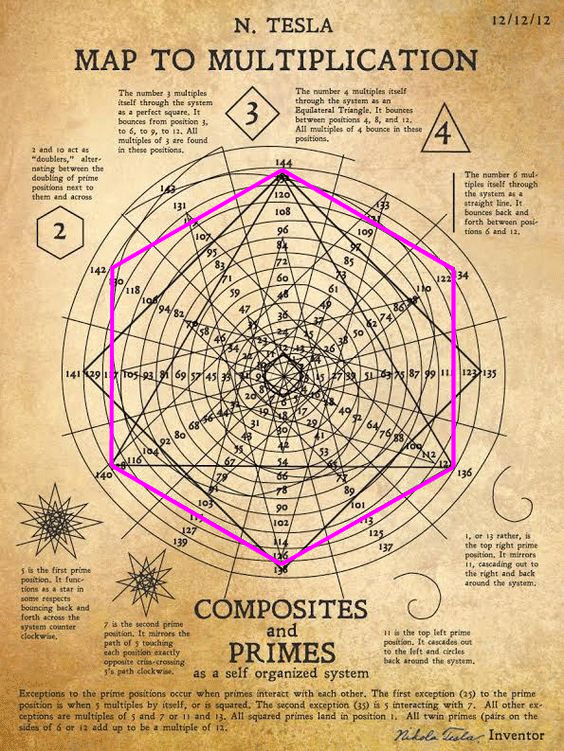This scheme has a configuration of 30 nodes so the recombination is involving 2x30 or 60 nodes out of the 72 nodes of True Prime Pairs will act as the base platform. The rest of 11 which is initially came out from the prime 13 is the irrational.

Note

The approach taken is to think of the solutions of an equation as a geometric object. For example, an equation in two variables defines a curve in the plane. More generally, an equation, or system of equations, in two or more variables defines a curve, a surface or some other such object in n-dimensional space (Wikipedia).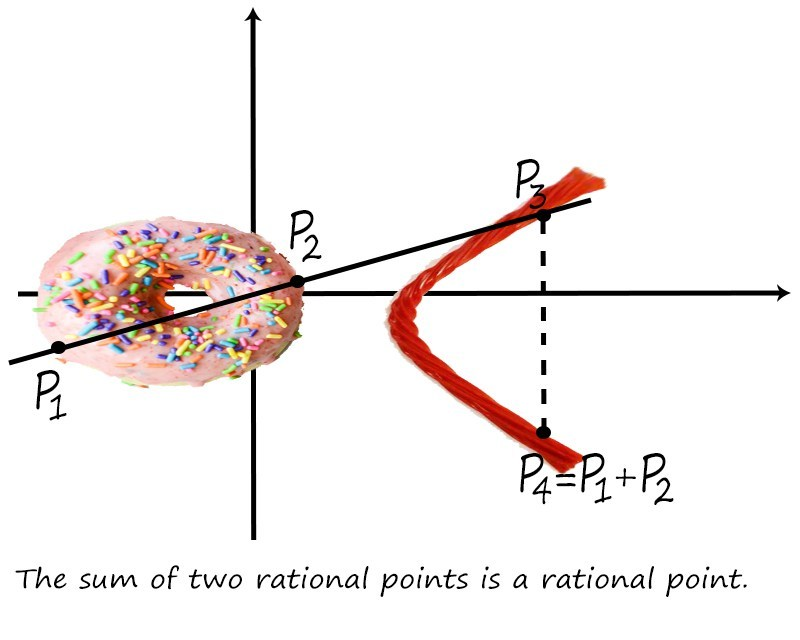Proceeding, the number line begins to coil upon itself; 20 lands on 2's cell, 21 on 3's cell. Prime number 23 sends the number line left to form the fourth hexagon, purple. As it is not a twin, the clockwise progression (rotation) reverses itself. When viewed with an extra dimension of space, these respectively generate hyperboloids of one sheet and two sheets.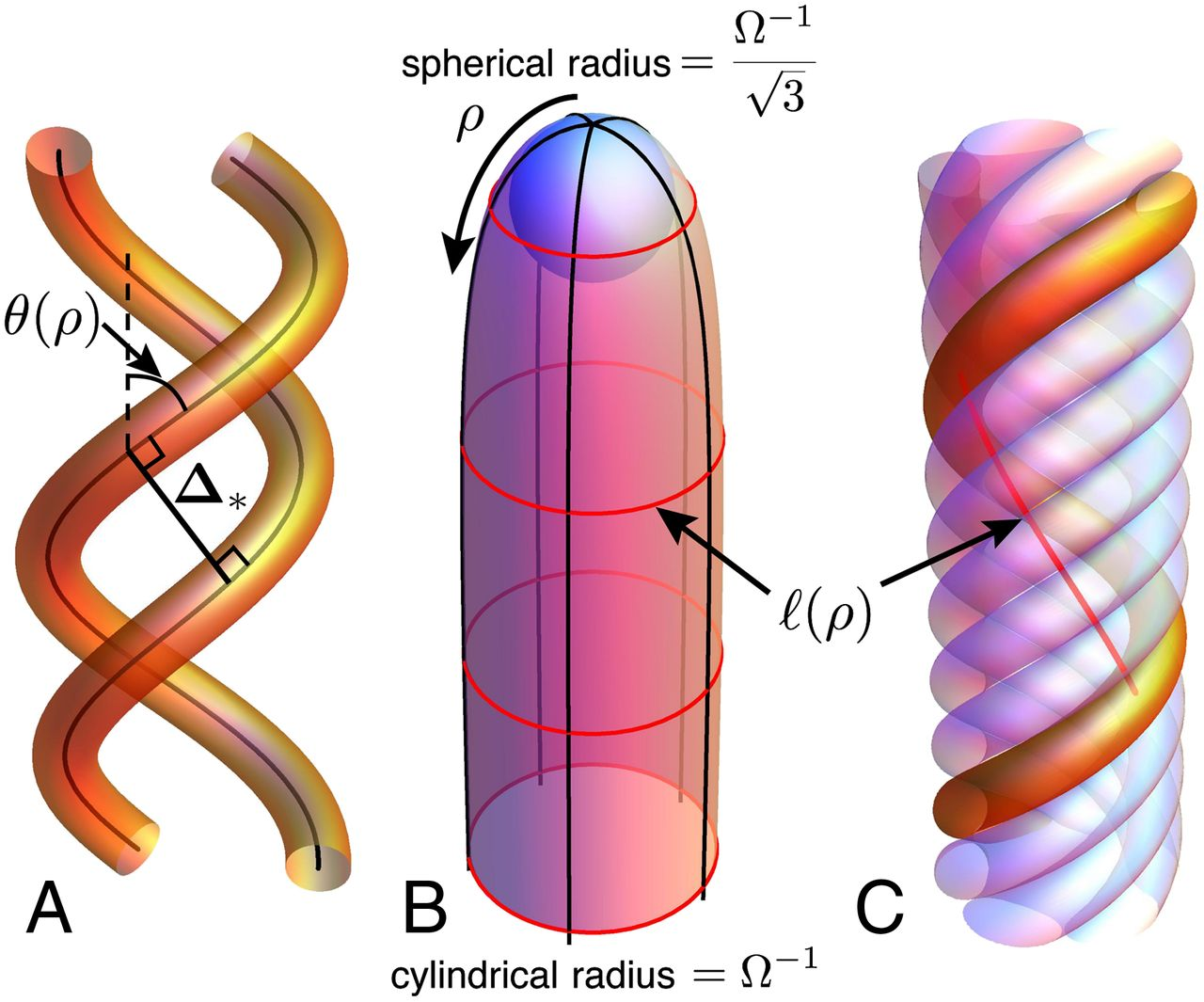The concept of dark matter arose in the study of cosmological phenomena, that is matters dealing with the Universe and galaxies and so on. However, evidence from the Hubble telescope in 1998 showed that the Universe began expanding at an accelerating rate sometime in the past and still is doing so. This came as a surprise to many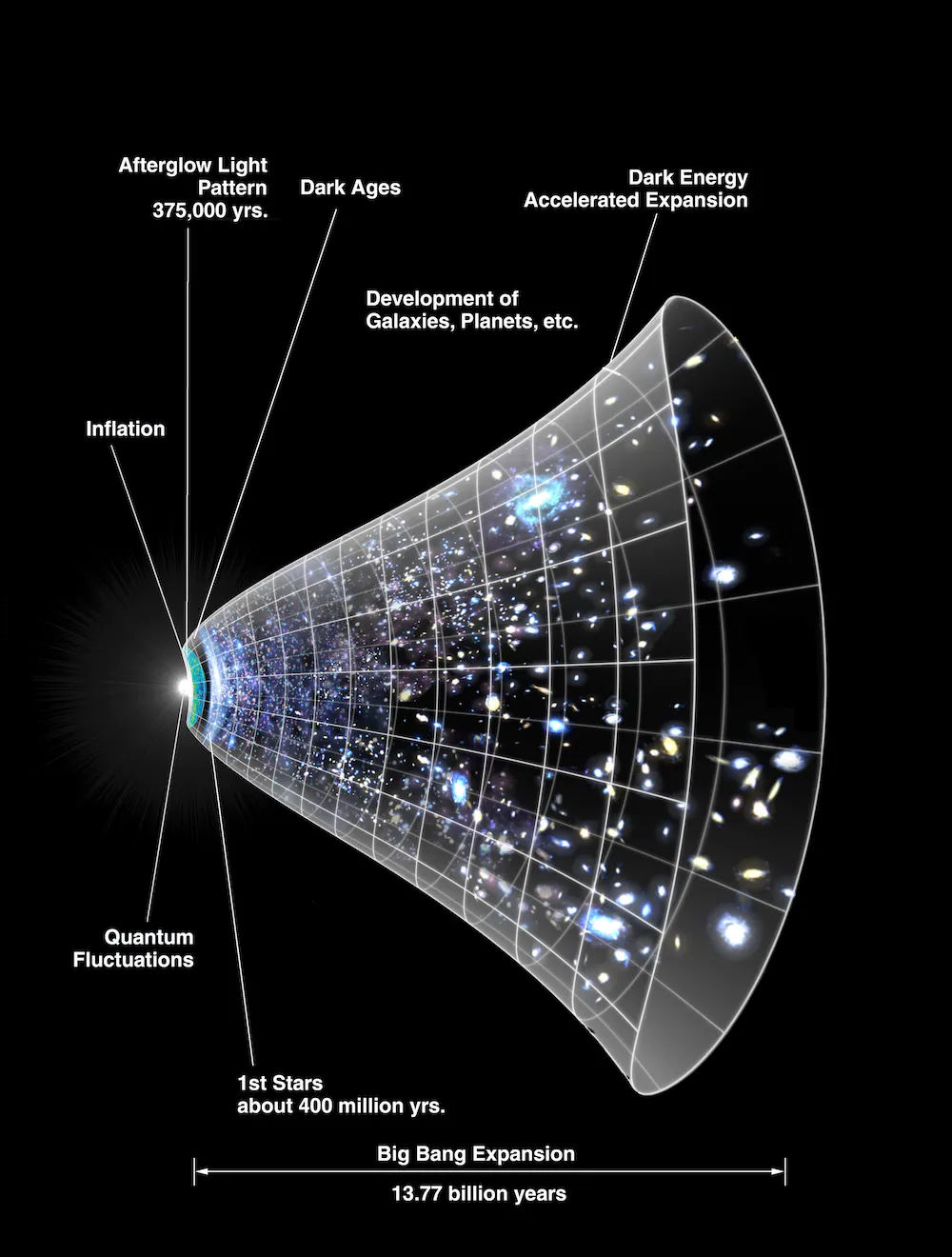The major problem, however, is that quantum mechanical calculations for the cosmological constant give value that is grossly out of the required range. This indicates that either something is wrong with the theory, or our knowledge is incomplete.